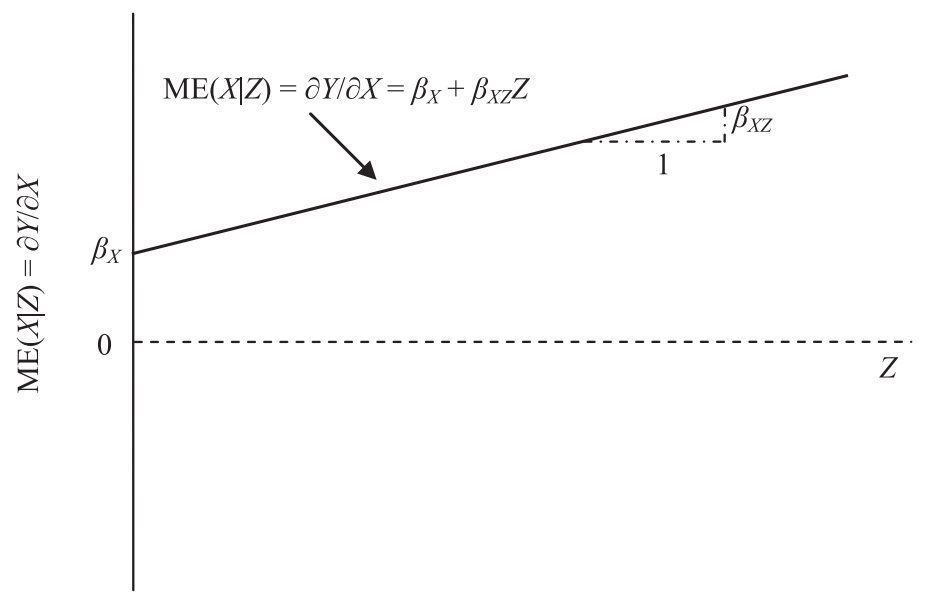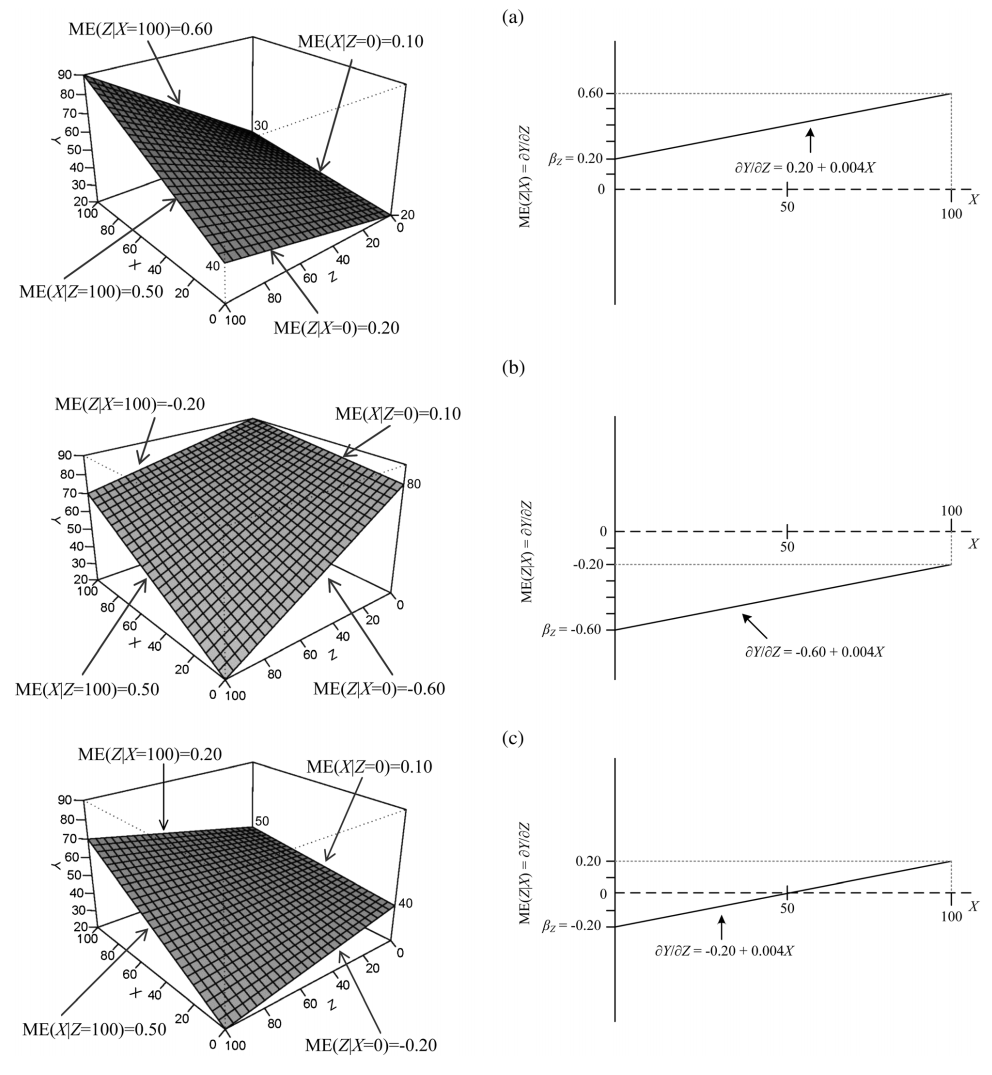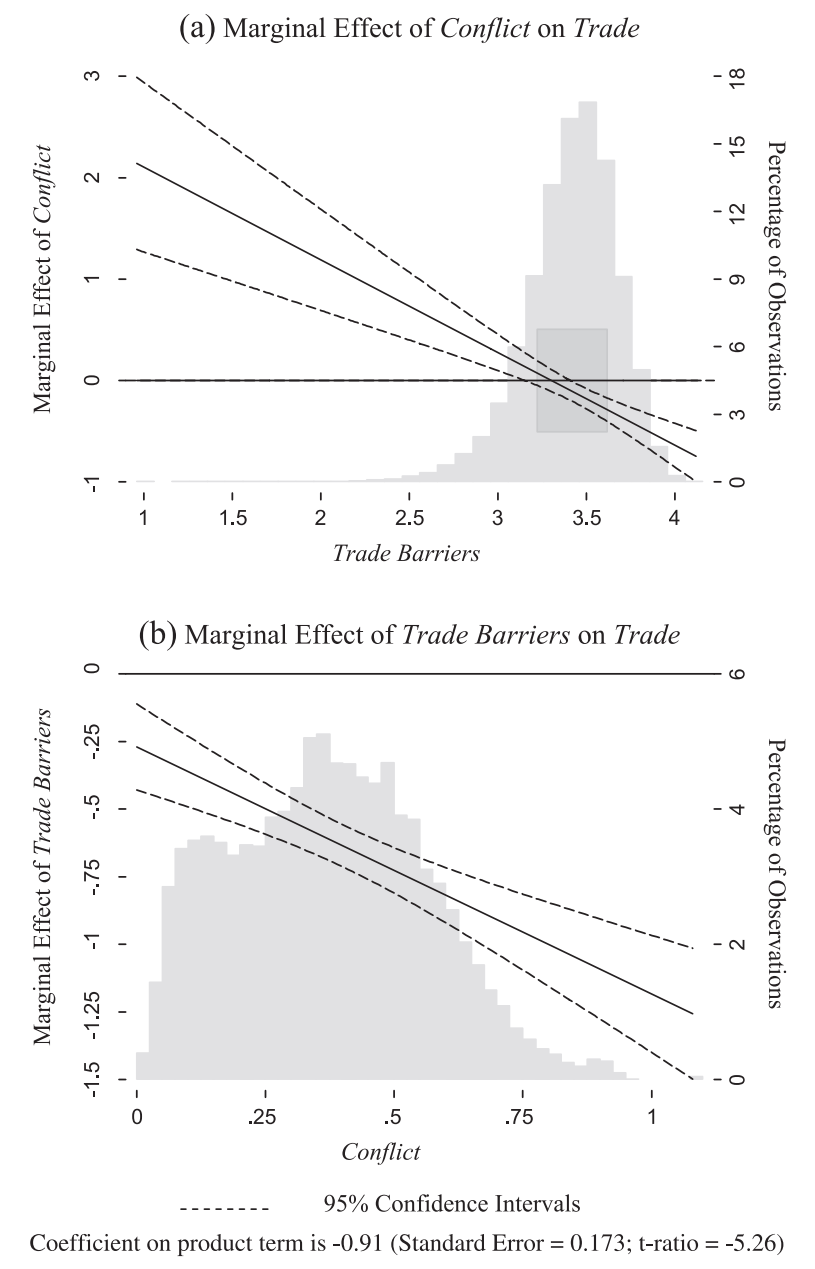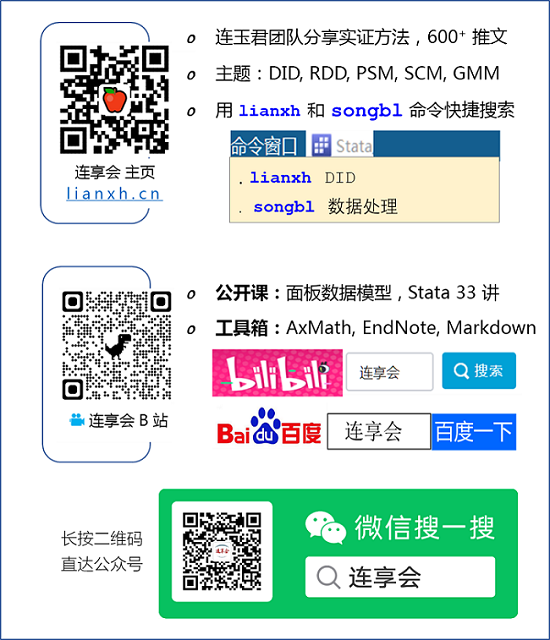# Stata：交乘项的对称效应与图示

Stata 连享会   主页 || 视频 || 推文

Source： Berry W D, Golder M, Milton D. Improving tests of theories positing interaction[J]. The Journal of Politics, 2012, 74(3): 653-671. -online appendix--replication files--webpage--pdf-

## 2. 边际效应图示

• 当 $Z=0$ 时，$X$ 对 $Y$ 的边际效应为 ${\beta }_{{}_{X}}$

• 随着 $Z$ 的增加，$X$ 对 $Y$ 的边际效应也逐渐增大。从数值上看，$Z$ 每增加一个单位，$X$ 对 $Y$ 的边际效应增加 ${\beta }_{XZ}$图 1：X 对 Y 的边际效应

## 3. 交互的对称性

$X$ 对 $Y$ 的边际效应是以 $Z$ 为条件的，反过来，也可以认为 $Z$ 对 $Y$ 的边际效应是以 $X$ 为条件的。具体来看，$Z$ 的边际效应可以写成下式：图 2：X、Z 和 Y 之间的条件关系

## 4. 改进建议

• 当 $Z$ 取最小值时，$X$ 的边际效应是 [正 负 零]；
• 当 $Z$ 取最大值时，$X$ 的边际效应是 [正 负 零]；
• 当 $X$ 取最小值时，$Z$ 的边际效应是 [正 负 零]；
• 当 $X$ 取最小值时，$Z$ 的边际效应是 [正 负 零]；
• $X$ 的边际效应 与 $Z$ 的关系是 [正 负]，反之亦然。

• 在所有 $Z$ 值处，$X$ 的边际效应均为正，并且这种效应在 $Z$ 值最小时最强，随着 $Z$ 的增加而减小；

• 在 $X$ 值最小时，$Z$ 对 $Y$ 的边际效应为正；随着 $X$ 的增加，这种效应在减小；在 $X$ 的某个值处，$Z$ 对 $Y$ 边际效应为零；当 $X$ 值进一步增加时，$Z$ 对 $Y$ 边际效应为负，并随 $X$ 的增加这种负向效应也在增加。

## 5. 论文重现

### 5.1 研究背景

Kastner (2007) 研究了利益冲突和具有国际经济利益主体的实力对国家间贸易水平的影响。以往的研究表明，当国家之间存在政治利益冲突时，双边贸易会降低，但不同国家又有很大差异。之所以如此，Kastner (2007) 认为尽管各国领导人都希望减少与与自己利益不一致国家的贸易，但这也会受到拥有国际经济利益的国内主体的限制。因此，我们可以认为，当具有国际经济利益的国内主体拥有强大政治影响力时，利益冲突对贸易影响会相对较小。

### 5.2 研究假设

• 当贸易壁垒强度最低时，利益冲突对贸易的边际效应为负；

• 当贸易壁垒强度最高时，利益冲突对贸易的边际效应为负；

• 利益冲突的边际效应与贸易壁垒负相关，反之也成立。

### 5.4 边际效应图示

• 无论利益冲突取何值，贸易壁垒对贸易的边际效应都是负的；并且，在利益冲突最小时，这种负面影响最弱；随着利益冲突增加，这种负面影响也加强。

• 当利益冲突最低时，贸易壁垒对贸易的边际效应为负；

• 当利益冲突最高时，贸易壁垒对贸易的边际效应为负。图 3：边际效应图示

### 5.5 附：绘图 Stata 代码

``````*数据地址
* https://sci-hub.se/10.1177/0022002707302804
*-or
* https://gitee.com/arlionn/data/blob/master/data01/TradeConflict.zip
*-Note: 请右击后另存，不要直接打开。

use TradeConflict.dta, clear
rename lnrtrade trade
rename logUNsun conflict
rename logAvBCFE tradebarriers
rename lnBlnUN conflict_tradebarriers

reg trade conflict tradebarriers conflict_tradebarriers          ///
laglnrtrade lnrgdpab lndist lnrpciab avremote landlocked       ///
island landratio pciratio jointdem, cluster(undirectedcode)

matrix b=e(b)
matrix V=e(V)
scalar b1=b[1,1]
scalar b2=b[1,2]
scalar b3=b[1,3]

scalar varb1=V[1,1]
scalar varb2=V[2,2]
scalar varb3=V[3,3]

scalar covb1b3=V[1,3]
scalar covb2b3=V[2,3]
scalar list b1 b2 b3 varb1 varb2 varb3 covb1b3 covb2b3

centile tradebarriers if e(sample), centile(20 80)
scalar p20=r(c_1)
scalar p80=r(c_2)
gen p20=p20
gen p80=p80

generate MVZ=((_n-1)/100)
replace  MVZ=. if _n>414 | _n<97
gen conbx=b1+b3*MVZ if _n<414
gen consx=sqrt(varb1+varb3*(MVZ^2)+2*covb1b3*MVZ) if _n<414

gen ax=1.96*consx
gen upperx=conbx+ax
gen lowerx=conbx-ax

generate MVX=((_n-1)/100)
replace  MVX=. if _n>110

gen conbz=b2+b3*MVX if _n<110
gen consz=sqrt(varb2+varb3*(MVX^2)+2*covb2b3*MVX) if _n<110

gen az=1.96*consz
gen upperz=conbz+az
gen lowerz=conbz-az

gen yline=0

graph twoway hist tradebarriers, width(0.1) percent color(gs14) yaxis(2)                        ///
||  rarea p20 p80 lowerx if lowerx>-0.51 & lowerx<0.51, horizontal sort color(gs12)           ///
||  line conbx   MVZ, clwidth(medium) clpattern(solid) clcolor(blue) clcolor(black) yaxis(1)  ///
||  line upperx  MVZ, clpattern(dash) clwidth(thin) clcolor(black)   ///
||  line lowerx  MVZ, clpattern(dash) clwidth(thin) clcolor(black)   ///
||  line yline  MVZ,  clwidth(thin) clcolor(black) clpattern(solid)  ///
||  ,xlabel(1 1.5 2 2.5 3 3.5 4,  nogrid labsize(2))     ///
ylabel(-1 0 1 2 3, axis(1) nogrid labsize(2))        ///
ylabel(0 3 6 9 12 15 18, axis(2) nogrid labsize(2))  ///
xscale(noline)                                       ///
yscale(noline alt)                                   ///
yscale(noline alt axis(2))                           ///
legend(off)                                          ///
yline(0, lcolor(black) lpattern(dash) lwidth(thin))  ///
xtitle("" , size(2.5)  )                             ///
ytitle("" , axis(2) size(2.5))                       ///
xsca(titlegap(2))                                    ///
ysca(titlegap(2))                                    ///
scheme(s2mono) graphregion(fcolor(white))

graph twoway hist conflict, width(0.025) percent color(gs14) yaxis(2)                  ///
|| line conbz   MVX, clwidth(medium) clpattern(solid) clcolor(blue) clcolor(black)   ///
|| line upperz  MVX, clpattern(dash) clwidth(thin) clcolor(black)                    ///
|| line lowerz  MVX, clpattern(dash) clwidth(thin) clcolor(black)                    ///
|| ,xlabel(0 .25 .5 .75 1, nogrid labsize(2))                           ///
ylabel(-1.5 -1.25 -1 -.75 -.5 -.25 0 , axis(1) nogrid labsize(2))   ///
ylabel(0 2 4 6, axis(2) nogrid labsize(2))                          ///
yscale(noline alt)                      ///
yscale(noline alt axis(2))              ///
xscale(noline)                          ///
legend(off)                             ///
yline(0, lcolor(black) lwidth(thin))    ///
xtitle("" , size(2.5)  )                ///
ytitle("" , axis(2) size(2.5)  )        ///
xsca(titlegap(2))                       ///
ysca(titlegap(2))                       ///
scheme(s2mono) graphregion(fcolor(white))
``````

### 5.6 结论

Kastner (2007) 论文的重现，表明了边际效应图在解释交互效应的重要性。尽管，Kastner (2007) 并没有解释为何「在贸易壁垒较低时，即具有国际经济利益的国内主体政治影响力强时，利益冲突对贸易的边际效应为正」，但是图 3b 进一步为其理论提供了证据。

## 6. 参考文献和扩展阅读

• Berry W D, Golder M, Milton D. Improving tests of theories positing interaction[J]. The Journal of Politics, 2012, 74(3): 653-671. -Link-
• Kastner S L. When do conflicting political relations affect international trade?[J]. Journal of Conflict Resolution, 2007, 51(4): 664-688. -Link-
• 专题: 回归分析
• 专题: 内生性-因果推断
• 专题: 交乘项-调节

## 相关课程

http://lianxh.duanshu.com

### 课程一览

Stata数据清洗 游万海 直播, 2 小时，已上线

Note: 部分课程的资料，PPT 等可以前往 连享会-直播课 主页查看，下载。

#### 关于我们

• Stata连享会 由中山大学连玉君老师团队创办，定期分享实证分析经验。直播间 有很多视频课程，可以随时观看。
• 连享会-主页知乎专栏，300+ 推文，实证分析不再抓狂。
• 公众号推文分类： 计量专题 | 分类推文 | 资源工具。推文分成 内生性 | 空间计量 | 时序面板 | 结果输出 | 交乘调节 五类，主流方法介绍一目了然：DID, RDD, IV, GMM, FE, Probit 等。
• 公众号关键词搜索/回复 功能已经上线。大家可以在公众号左下角点击键盘图标，输入简要关键词，以便快速呈现历史推文，获取工具软件和数据下载。常见关键词：`课程, 直播, 视频, 客服, 模型设定, 研究设计, stata, plus, 绘图, 编程, 面板, 论文重现, 可视化, RDD, DID, PSM, 合成控制法`连享会主页 lianxh.cn

✏ 连享会学习群-常见问题解答汇总：
https://gitee.com/arlionn/WD